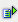The function int (num) returns the next smaller whole-numbered value. Negative values are rounded from 0 away.

Syntax: =int(num)Example =int(2.2) is 2 =int(-2,2) is -3